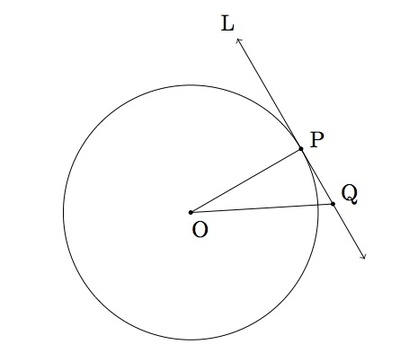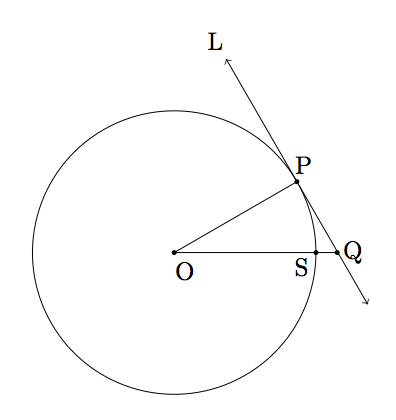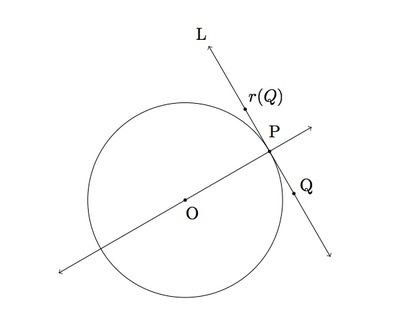# Tangent Lines and the Radius of a Circle

Alignments to Content Standards: G-C.A.2 G-CO.C.9 G-CO.A

Consider a circle with center $O$ and let $P\,$ be a point on the circle. Suppose $L$ is a tangent line to the circle at $P$, that is $L$ meets the circle only at $P$.Show that $\overline{OP}\,$ is perpendicular to $L$.

## IM Commentary

This task presents a foundational result in geometry, presented with deliberately sparse guidance in order to allow a wide variety of approaches. Teachers should of course feel free to provide additional scaffolding to encourage solutions or thinking in one particular direction. We include three solutions which fall into two general approaches, one based on reference to previously-derived results (e.g., the Pythagorean Theorem), and another conducted in terms of the geometry of rigid transformations. The foundational nature of the task likely renders the task inappropriate for assessment.

Commentary on Approach 1 (G-C.2, G-CO.9)

A first approach to this problem is to reason directly from past results in plane geometry. In the first solution, we reference in particular the fact that if $A$ is a point not a line $M$ and $B$ is the closest point on $M$ to $A$ then segment $\overline{AB}$ meets $M$ perpendicularly. (If so desired, a task devoted to discovering that relationship is presented in the task with name ''Shortest segment from a point $P\,$ to a line $L$.'') An appealing aspect of this solution is that it requires close focus on the definition of a circle. If $O$ is the center of a circle of radius $r$, then points of the plane fall into one of three categories:

• Points $Q$ so that $|QO| \lt r$: this is what is usually called the interior of the circle.
• Points $Q$ so that $|QO| = r$: these are the points on the circle.
• Points $Q$ so that $|QO| \gt r$: this is what is usually called the exterior of the circle.

By assumption the tangent line to the circle at $P\,$ contains only one point from the second category above. The essence of the argument presented here is that the tangent line in question cannot contain any points from the first category because this would force the line to meet the circle in a second point.

The second solution under this approach fills in some of the details of the first solution. In particular, it uses the Pythagorean Theorem and a proof by contradiction to establish that the tangent line and radius meet perpendicularly. The teacher may wish to go over the logic of arguments by contradiction separately and make sure the students are comfortable with this logic.

Commentary on Approach 2 (G-C.2, G-CO.A)

A second approach to the problem at hand is to use the idea of rigid motions of the plane. The argument here is independent of the Pythagorean theorem (which is required in the previous approach) and shows some of the power of the theory of rigid motions.

The instructor may wish to recall visually the effect of reflections of the plane as well as the mathematical definition: reflection of a point $P\,$ about a line $L$ sends $P\,$ to the point $Q$ so that $L$ is the perpendicular bisector of segment $\overline{PQ}$. This definition applies to points $P\,$ that are not on $L$: if $P\,$ is on $L$ then the reflection of $P\,$ about $L$ is $P$. Students may be encouraged to experiment with some different lines, including the tangent line in question, to develop an intuition. After the experimenting, students should have a sense that the only lines which are preserved under this reflection are $L$ itself and those lines which are perpendicular to $L$. It is vital that they have a firm grasp of this since the logic of this argument is to use this fact in order to conclude that $L$ is perpendicular to segment $\overline{OP}$.

Finally, we note that the transformation-based solution provided explicitly uses the fact that there is a unique tangent line to a circle at any given point, so instructors should either assume this knowledge or supplement the current task with the task "Uniqueness of the tangent line to a circle.''

## Solutions

Solution: 1 (G-C.A.2, G-CO.C.9)

We argue from the fact that the shortest segment from a point $P\,$ not on $L$ to a line $L$ is perpendicular to $L$. It remains only to argue that the radius $\overline{OP}\,$ is the shortest line segment from $O$ to the line $L$.Suppose $Q$ is some other point, an example of which is pictured above, not equal to $P\,$ on line $L$. We first observe that we cannot have $|QO| \lt |OP|$, since this would mean some points on line $L$ lie inside the circle, i.e., that $L$ contains some chord $\overline{PS}$ of the circle. But this can't be, since we've assumed that $L$ is a tangent line, and so meets the circle only once (and not at a second point $S$). Next, we note that we cannot have $|QO| = |PO|$ or else $L$ would meet the circle in the two distinct points $P\,$ and $S$. The only other possibility remaining is that $|OQ| \gt |OP|$. Since this is true for all points $Q \neq P\,$ on $L$ we conclude that $P\,$ is the closest point on $L$ to $O$ and so $\overline{OP}$ is the shortest line segment joining $O$ to a point on $L$, as desired.

Solution: 2 (G-C.A.2, G-CO.C.9)

This is a more detailed version of the previous solution, where (a) we argue more carefully that the point $P$ is the closest point of $L$ to $O$, and then (b) use the Pythagorean Theorem to establish that $L$ is perpendicular to the radius $\overline{OP}$.

1. Pictured below is a point $Q$, different from $P$, on the line $L$:The ray $\overrightarrow{OQ}$ meets the circle in exactly one point which we will have labelled $S$. We know that $Q \neq S$ because $L$ meets the circle only at the point $P$. If $Q$ is between $S$ and $O$ this means that $|OQ| \lt |OS| = |OP|$ and so the chord which contains $P$ and $Q$ is part of the line $L$. This is not possible, however, because $L$ only meets the circle at the point $P$. So it must be that $S$ is between $O$ and $Q$ and we have

\begin{array} .|OQ| &= |OS| + |SQ| \\ &\gt |OS| \\ &= |OP| \end{array}
2. Below is a picture of the line $L$ and the points $O$ and $P$ in a case where segment $\overline{OP}$ is not perpendicular to $L$: we have removed the circle from the picture.Also pictured is the point $R$ so that segment $\overline{OR}$ is perpendicular to $L$. Since angle $\angle ORP$ is a right angle we can apply the Pythagorean theorem to conclude that $$|OP|^2 = |OR|^2 + |PR|^2.$$ It follows that $|OP|^2 \gt |OR|^2$ and so $|OR|$ is less than $|OP|$.

Now from before, we know that if $Q$ is any point on $L$ other than $P$ then $$|OQ| \gt |OP|.$$ Now from above, if $\overline{OP}$ were not perpendicular to $L$ then there would be a point $R$ on $L$ which is closer to $O$ than $P$ that is $$|OR| \lt |OP|.$$ Setting $Q = R$ in the previous equation, these two equation are not both possible. Since the hypothesis that $\overline{OP}$ is not perpendicular to $L$ led to a contradiction, it must be that $\overline{OP}$ is in fact perpendicular to $L$.

Solution: 3 (G-C.A.2, G-CO.A)

We consider the reflection $r$ over the line $\overleftrightarrow{OP}$ containing the diameter of the circle through $P$. In the picture below are a point $Q$ on $L$ other than $P$, and its reflection $r(Q)$. Note that since they lie on the diameter itself, we have $r(O)=O$ and $r(P)=P$. We argue that as illustrated, the reflection of $Q$ must lie on $L$, and more generally that $r(L)=L$.Let $R$ be the radius of the circle. Since reflections preserve distances, we know that for a point $Q$ on $L$, we have $R=|QO|$ if and only if $R=|r(Q)O|$, i.e., $Q$ is on the circle if and only if $r(Q)$ is. Thus since $P$ is the only point of $L$ on the circle, it is also the only point on $L$ whose reflection is on the circle. This means that $r(L)$ intersects the circle at precisely the one point $r(P)=P$, and hence $r(L)$ is a tangent line to the circle at $P$. By the uniqueness of the tangent line at $P$, we conclude that $r(L)=L$.

Now if $Q$ is any point on $L$ other than $P$, then the axis of reflection $\overleftrightarrow{OP}$ must be perpendicular to the line $\overleftrightarrow{Qr(Q)}$. But since $r(Q)$ is on $L$ (since it is on $r(L)$, and we have just shown that $r(L)=L$), we have that $\overleftrightarrow{Qr(Q)}=L$, and hence conclude that the radius $\overline{OP}$ is indeed perpendicular to $L$.